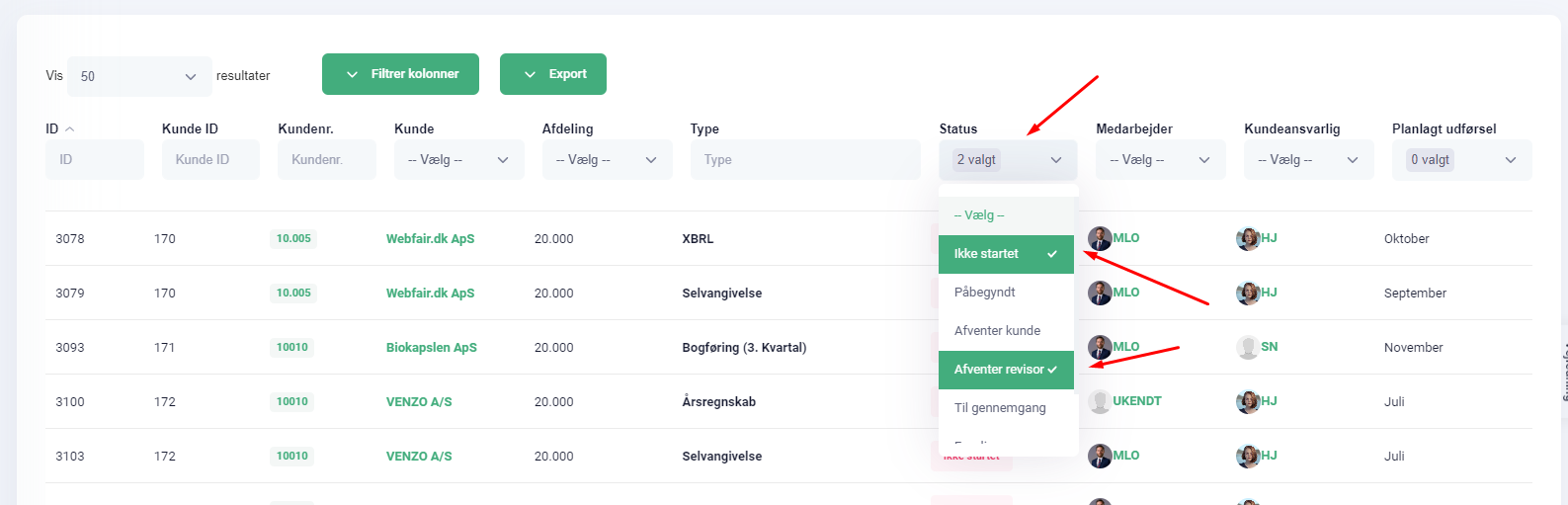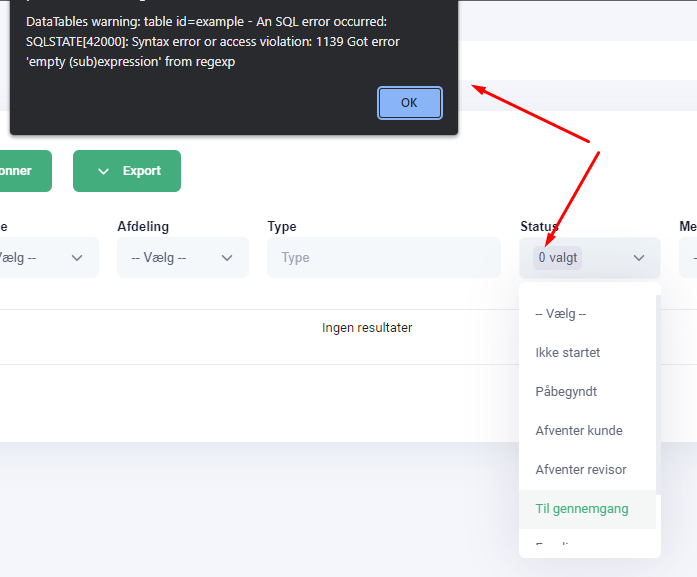# Server Side REGEX searchThis is my columns and I'm trying to use 'search' function to REGEX those values in Status column. It works just fine choosing them and the REGEX actually sorts and filter correctly.

My problem is that when I do unselect all values, I get this message:Now the REGEX in my AJAX function is like:
api.column(colIdx).search('(^\$|' + search_selected_val + ')',true,false).draw();

And my Server Side SSP script processes the REGEX this way:
if(\$regexp){
\$binding = self::bind( \$bindings, \$str, PDO::PARAM_STR );
\$columnSearch[] = "`".\$column['db']."` REGEXP ".\$binding;
} else {
\$binding = self::bind( \$bindings, '%'.\$str.'%', PDO::PARAM_STR );
\$columnSearch[] = "`".\$column['db']."` LIKE ".\$binding;
}

Anyone who know where this is wrong?• This is my whole AJAX script for REGEX:

`\$('select', \$('.filters th').eq(\$(api.column(colIdx).header()).index())).off('change').on('change', function (e) {
e.stopPropagation();

``````                    var selected_val = \$(this).select2('data');
var search_selected_val = '';
for (i = 0; i < selected_val.length; ++i) {
if(i > 0) {
search_selected_val += '|';
}
search_selected_val += selected_val[i].text;
}

if(search_selected_val == '-- Vælg --') {
search_selected_val = '';
}

check_related_1 = colIdx;
check_related_2 = \$(this).parent().parent().parent();
check_related_3 = search_selected_val;

api.column(colIdx).search('(^\$|' + search_selected_val + ')',true,false).draw();
checkRelated(colIdx, \$(this).parent().parent().parent(), search_selected_val);

});`
``````
• This is my filter class in SSP:

``````static function filter ( \$request, \$columns, &\$bindings )
{
\$globalSearch = array();
\$columnSearch = array();
\$dtColumns = self::pluck( \$columns, 'dt' );

if ( isset(\$request['search']) && \$request['search']['value'] != '' ) {
\$str = \$request['search']['value'];
\$regexp = \$request['search']['regex'];

for ( \$i=0, \$ien=count(\$request['columns']) ; \$i<\$ien ; \$i++ ) {
\$requestColumn = \$request['columns'][\$i];
\$columnIdx = array_search( \$requestColumn['data'], \$dtColumns );
\$column = \$columns[ \$columnIdx ];

if ( \$requestColumn['searchable'] == 'true' ) {
if(!empty(\$column['db'])){
if(\$regexp){
\$binding = self::bind( \$bindings, \$str, PDO::PARAM_STR );
\$globalSearch[] = "`".\$column['db']."` REGEXP ".\$binding;
} else {
\$binding = self::bind( \$bindings, '%'.\$str.'%', PDO::PARAM_STR );
\$globalSearch[] = "`".\$column['db']."` LIKE ".\$binding;
}
}
}
}
}

// Individual column filtering
if ( isset( \$request['columns'] ) ) {
for ( \$i=0, \$ien=count(\$request['columns']) ; \$i<\$ien ; \$i++ ) {
\$requestColumn = \$request['columns'][\$i];
\$regexp = \$requestColumn['search']['regex'];
\$columnIdx = array_search( \$requestColumn['data'], \$dtColumns );
\$column = \$columns[ \$columnIdx ];

\$str = \$requestColumn['search']['value'];

if ( \$requestColumn['searchable'] == 'true' && \$str != '' ) {
if(!empty(\$column['db'])){
if(\$regexp){
\$binding = self::bind( \$bindings, \$str, PDO::PARAM_STR );
\$columnSearch[] = "`".\$column['db']."` REGEXP ".\$binding;
} else {
\$binding = self::bind( \$bindings, '%'.\$str.'%', PDO::PARAM_STR );
\$columnSearch[] = "`".\$column['db']."` LIKE ".\$binding;
}
}
}
}
}

// Combine the filters into a single string
\$where = '';

if ( count( \$globalSearch ) ) {
\$where = '('.implode(' OR ', \$globalSearch).')';
}

if ( count( \$columnSearch ) ) {
\$where = \$where === '' ?
implode(' AND ', \$columnSearch) :
\$where .' AND '. implode(' AND ', \$columnSearch);
}

if ( \$where !== '' ) {
\$where = 'WHERE '.\$where;
}

return \$where;
}
``````